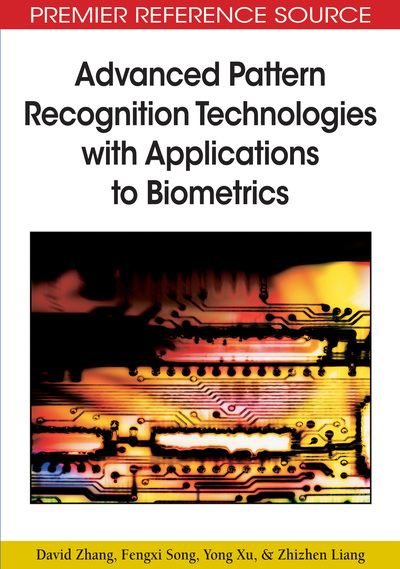# Tensor Space

David Zhang (Hong Kong Polytechnic University, Hong Kong), Fengxi Song (New Star Research Institute Of Applied Technology, China), Yong Xu (Harbin Institute of Technology, China) and Zhizhen Liang (Shanghai Jiao Tong University, China)
DOI: 10.4018/978-1-60566-200-8.ch007
Available
\$37.50
No Current Special Offers

## Abstract

In this chapter, we first give the background materials for developing tensor discrimination technologies in Section 7.1. Section 7.2 introduces some basic notations in tensor space. Section 7.3 discusses several tensor decomposition methods. Section 7.4 introduces the tensor rank.
Chapter Preview
Top

## Background

Matrix decompositions, such as the singular value decomposition (SVD), are ubiquitous in numerical analysis. One usual way to think of the SVD is that it decomposes a matrix into a sum of rank-1 matrices. In other words, anmatrix A can be expressed as a minimal sum of rank-1 matrices:A =, (7.1) whereandfor all. The operatordenotes the outer product. Thus the ijth entry of the rank-1 matrixis the product of the ith entry of a and the jth entry of b, denoted by.Such decompositions provide possibilities to develop fundamental concepts such as the matrix rank and the approximation theory and gain a range of applications including WWW searching and mining, image processing, signal processing, medical imaging, and principal component analysis. The decompositions are well-understood mathematically, numerically, and computationally.

A tensor is a higher order generalization of a vector or a matrix. In fact, a vector is a first-order tensor and a matrix is a tensor of order two. Furthermore speaking, tensors are multilinear mapping over a set of vector spaces. If we have data in three or more dimensions, then we mean to deal with a higher-order tensor. In tensor analysis, higher-order tensor (also known as multidimensional, multiway, or n-way array) decompositions (Martin, 2004; Comon, 2002) are used in many fields and also have received considerable theoretical interest.

Different from some classical matrix decompositions, extending matrix decompositions such as the SVD to higher-order tensors has proven to be quite difficult. Familiar matrix concepts such as rank become ambiguous and more complicated. One goal of the tensor decomposition is the same as for a matrix decomposition: to rewrite the tensor as a sum of rank-1 tensors. Consider, for example, antensor A. We would like to express A as the sum of rank-1 third-order tensors, that is,A =, (7.2) where,,, andfor all.Note that if a, b, c are vectors, then.

### Basic Notations

In this section, we introduce some elementary notations and definitions needed in the later chapter.

## Complete Chapter List

Search this Book:
Reset